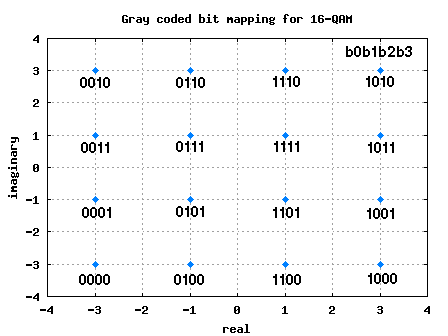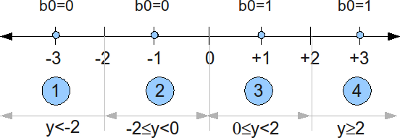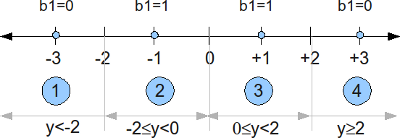- DSP log - http://www.dsplog.com -

Softbit for 16QAM

Posted By Krishna Sankar On July 5, 2009 @ 10:05 pm In Modulation | 34 Comments

In the post on Soft Input Viterbi decoder , we had discussed BPSK modulation with convolutional coding and soft input Viterbi decoding in AWGN channel. Let us know discuss the derivation of soft bits for 16QAM modulation scheme with Gray coded bit mapping. The channel is assumed to be AWGN alone.

## Gray Mapped 16-QAM constellation

In the past, we had discussed BER for 16QAM in AWGN  modulation. The 4 bits in each constellation point can be considered as two bits each on independent 4-PAM modulation on I-axis and Q-axis respectively.

 b0b1 I b2b3 Q 00 -3 00 -3 01 -1 01 -1 11 +1 11 +1 10 +3 10 +3

Table: Gray coded constellation mapping for 16-QAMFigure: 16QAM constellation plot with Gray coded mapping

## Channel Model

, where

is the modulated coded sequence taking values in the alphabet

.

is the Additive White Gaussian Noise following the probability distribution function,

with mean and variance .

## Demodulation

For demodulation, we would want to maximize the probability that the bit was transmitted given we received i.e . This criterion is called Maximum a posteriori probability (MAP).

Using Bayes rule ,

.

Note: The probability that all constellation points occur are equally likely, so maximizing is equivalent to maximizing .

## Soft bit for b0

The bit mapping for the bit b0 with 16QAM Gray coded mapping is shown below. We can see that when b0 toggles from 0 to 1, only the real part of the constellation is affected.Figure: Bit b0 for 16QAM Gray coded mapping

When the b0 is 0, the real part of the QAM constellation takes values -3 or -1. The conditional probability of the received signal given b0 is 0 is,

.

When the bit0 is 1, the real part of the QAM constellation takes values +1 or +3. The conditional probability given b0 is zero is,

.

We can define a likelihood ratio that if

.

The likelihood ratio for b0 is,

.

### Region #1 ()

When , then we can assume that relative contribution by constellation +3 in the numerator and -1 in the denominator is less and can be ignored. So the likelihood ratio reduces to,

.

Taking logarithm on both sides,

.

### Region #2 (), Region #3 ()

When or , then we can assume that relative contribution by constellation +3 in the numerator and -3 in the denominator is less and can be ignored. So the likelihood ratio reduces to,

.

Taking logarithm on both sides,

.

### Region #4 ()

If , then we can assume that relative contribution by constellation +1 in the numerator and -3 in the denominator is less and can be ignored. So the likelihood ratio reduces to,

.

Taking logarithm on both sides,

.

## Soft bit for b1

The bit mapping for the bit b1 with 16QAM Gray coded mapping is shown below. We can see that when b0 toggles from 0 to 1, only the real part of the constellation is affected.Figure: Bit b1 for 16QAM Gray coded mapping

When the b1 is zero, the real part of the QAM constellation takes values -3 or +3. The conditional probability given b1 is zero is,

.

When the bit0 is 1, the real part of the QAM constellation takes values -1 or +1. The conditional probability given b1 is one is,

.

We can define a likelihood ratio that if

.

The likelihood ratio for b1 is,

.

### Region #1 (), Region#2 ()

When or , then we can assume that relative contribution by constellation +1 in the numerator and +3 in the denominator is less and can be ignored. So the likelihood ratio reduces to,

.

Taking logarithm on both sides,

.

### Region #3 (), Region #4 ()

If or , then we can assume that relative contribution by constellation -1 in the numerator and -3 in the denominator is less and can be ignored. So the likelihood ratio reduces to,

.

Taking logarithm on both sides,

.

## Summary

Note: As the factor  is common to all the terms, it can be removed.

The softbit for bit b0 is,

.

The softbit for bit b1 is,

.

The softbit for bit b1 can be simplified to,

.

It is easy to observe that the softbits for bits b2, b3 are identical to softbits for b0, b1 respectively except that the decisions are based on the imaginary component of the received vector .

The softbit for bit b2 is,

.

The softbit for bit b3 is,

.

and

,

This simplification avoids the need for having a threshold check in the receiver for sofbits b0 and b2 respectively.

## Reference

URL to article: http://www.dsplog.com/2009/07/05/softbit-16qam/

URLs in this post:

 Soft Input Viterbi decoder: http://www.dsplog.com/2009/01/14/soft-viterbi/

 BER for 16QAM in AWGN: http://www.dsplog.com/2008/06/05/16qam-bit-error-gray-mapping/

 Bayes rule: http://en.wikipedia.org/wiki/Bayes%27_theorem#Statement

 Simplified Soft-Output Demapper for Binary Interleaved COFDM with Application to HIPERLAN/2, Filippo Tosato1, Paola Bisaglia, HPL-2001-246 October 10th , 2001,: http://www.hpl.hp.com/techreports/2001/HPL-2001-246.pdf